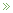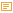• ## A PCA Puzzle

Posted by Konstantin 16.01.2012 No Comments

This post presumes you are familiar with PCA.

Consider the following experiment. First we generate a random vector (signal) as a sequence of random 5-element repeats. That is, something like

(0.5, 0.5, 0.5, 0.5, 0.5,   0.9, 0.9, 0,9, 0.9, 0,9,   0.2, 0.2, 0.2, 0.2, 0.2,   ... etc ... )

In R we could generate it like that:

```num_steps = 50
step_length = 5;
initial_vector = c();
for (i in 1:num_steps) {
initial_vector = c(initial_vector, rep(runif(1), step_length));
}```

Here's a visual depiction of a possible resulting vector:

Next, we shall create a dataset, where each element will be a randomly shifted copy of this vector:

```library(magic) # Necessary for the shift() function
dataset = c()
for (i in 1:1000) {
shift_by = floor(runif(1)*num_steps*step_length) # Pick a random shift
new_instance = shift(initial_vector, shift_by)   # Generate a shifted instance
dataset = rbind(dataset, new_instance);          # Append to data
}```

Finally, let's apply Principal Component Analysis to this dataset:

`pca = prcomp(dataset)`

Question - how do the top principal components look like? Guess first, then read below for the correct answer.

Tags: , ,

### Calendar

January 2012
M T W T F S S
« Dec   May »
1
2345678
9101112131415
16171819202122
23242526272829
3031